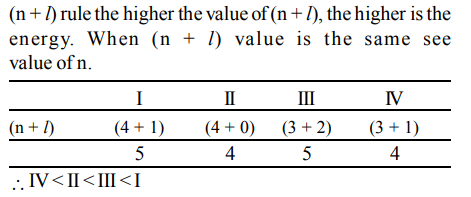## Atomic Structure Questions and Answers Part-10

1. The four quantum numbers of the valence electron of potassium are
a) 4, 1, 1, 1/2
b) 4, 0, 0, 1/2
c) 4, 5, 0, 1/2
d) 4, 4, 0, 1/2

Explanation: Valence electron of K is 4s1
n = 4, l = 0, m = 0, s = ½

2. The electrons, identified by quantum numbers n and l (i) n = 4, l = 1 (ii) n = 4, l = 0 (iii) n = 3, l = 2 (iv) n = 3, l = 1 can be placed in order of increasing energy, from the lowest to highest, as
a) (iv) < (ii) < (iii) < (i)
b) (ii) < (iv) < (i) < (iii)
c) (i) < (iii) < (ii) < (iv)
d) (iii) < (i) < (iv) < (ii)

Explanation:3. Bohr's model of atom is in conflict with
a) Pauli's exclusion principle
b) Heiseinberg's uncertainity principle
c) Max Planck's quantum theory
d) All the above three

Explanation: According to Bohr's model electrons follow definite path

4. What is the maximum number of electrons that can be accomodated in an atom in which the highest principal quantum number value is 4?
a) 10
b) 18
c) 36
d) 54

Explanation: Energy of subshells follow the order 1s2 , 2s2 , 2p6 , 3s2 , 3p6 , 4s2 , 3d10, 4p6 , 5s, 4d, 5p, 6s..... we can fill them by electrons upto 4p hence 36 electrons.

5. The total number of orbitals possible for principal quantum number n is
a) n
b) $n^{2}$
c) 2n
d) $2n^{2}$

Explanation: Total number iof orbitals for principal quantum number n is n2

6. Which of the following is not possible ?
a) n = 3, l = 0, m = 0
b) n = 3, l = 1, m = –1
c) n = 2, l = 0, m = –1
d) n = 2, l = 1, m = 0

Explanation: For l = 0, m cannot be –1

7. For how many orbitals, the quantum numbers n = 3, l =2, m = +2 are possible?
a) 1
b) 2
c) 3
d) 4

Explanation: m = +2 signifies only one orbital

8. The correct set of quantum numbers for a 4d electron is
a) $4,3,2,+\frac{1}{2}$
b) 4, 2, 1, 0
c) $4,3,-2,+\frac{1}{2}$
d) $4,2,1,-\frac{1}{2}$

Explanation: For 4d electron we have n = 4, l = 2, m = –2, –1, 0, +1, +2 & s = ½ or –½

9. For a, f-orbital, the values of m are
a) –2, –1, 0, +1, +2
b) –3, –2, –1, 0, +1, +2, 3
c) –1, 0, +1
d) 0, +1, +2, +3

10. The values of Planck's constant is $6.63 × 10^{-34}Js$   . The velocity of light is $3.0 × 10^{8}m s^{-1}$   . Which value is closest to the wavelength in nanometres of a quantum of light with frequency of $8 × 10^{15} s^{-1}$  ?
a) $5 × 10^{-18}$
b) $4 × 10^{1}$
c) $3 × 10^{7}$
d) $2 × 10^{-25}$